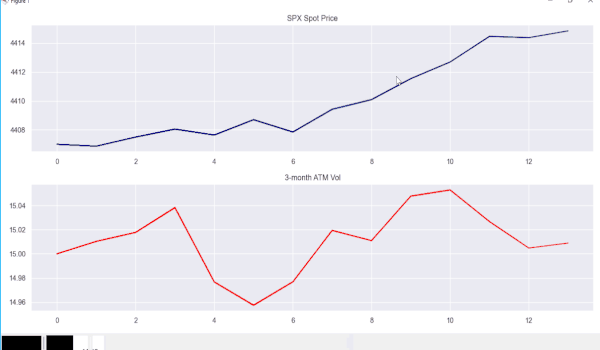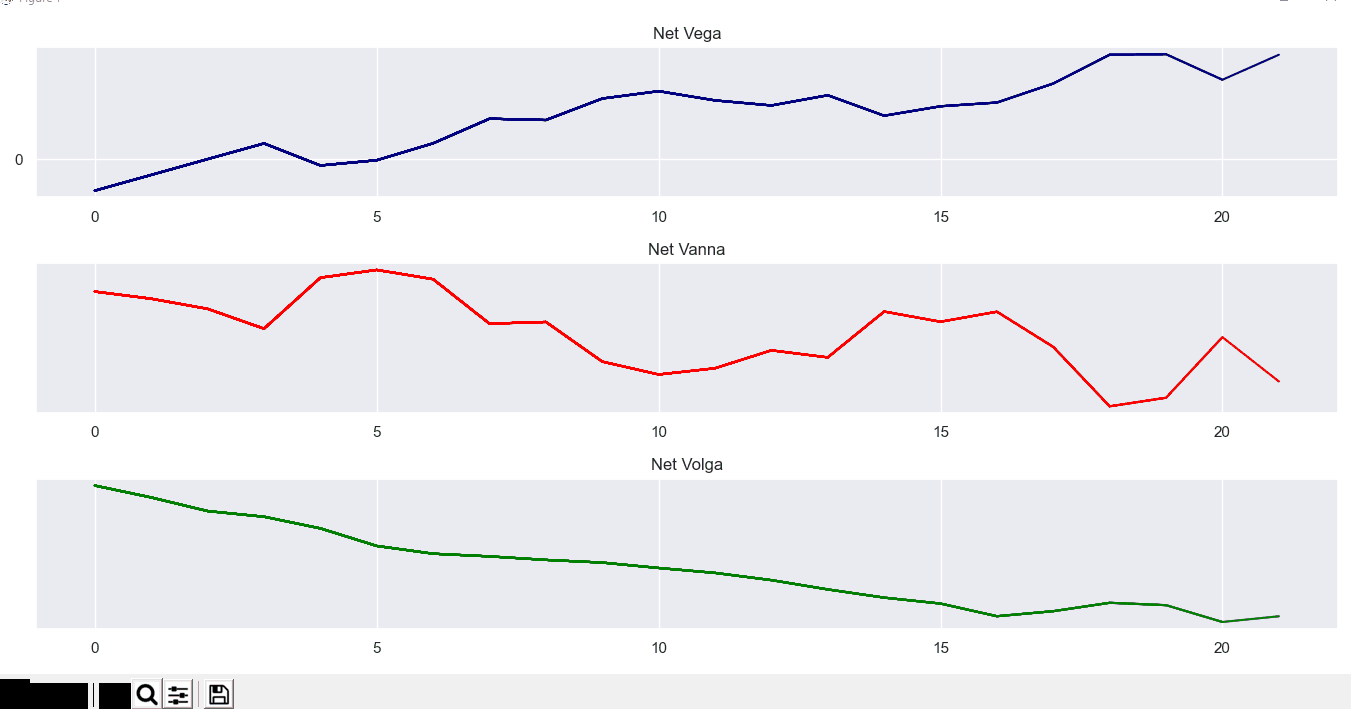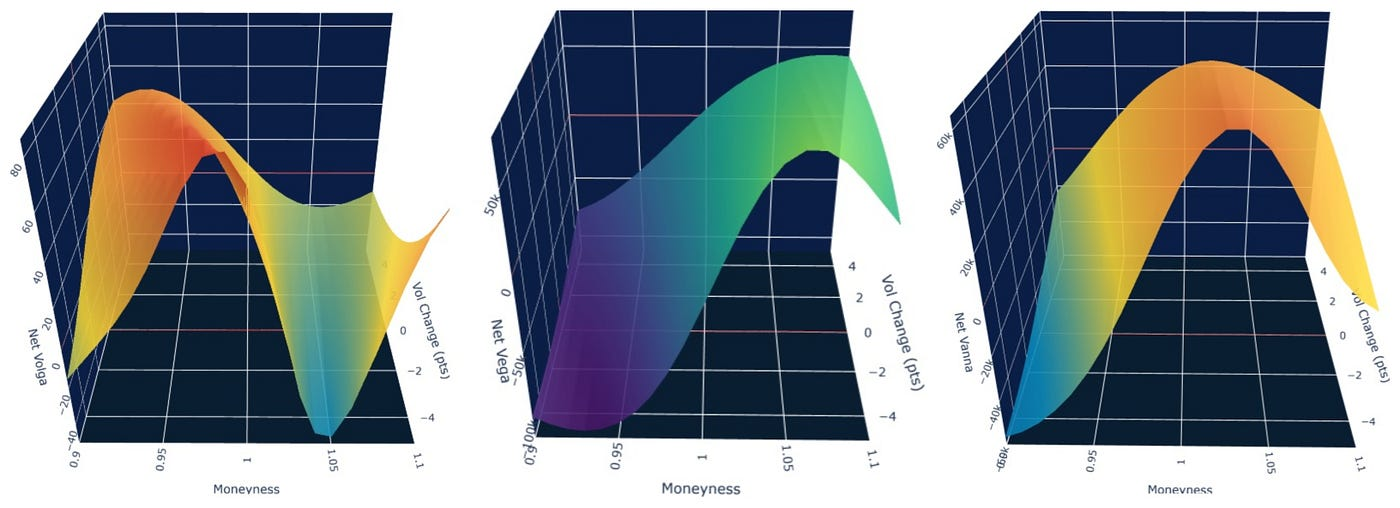# Volatility Smile and Delta Hedging (Part 1) — Getting intimate with the vol surface.

1. Flat volatility across all strikes
2. Curved volatility smile corresponded with the same strikes (where we assume that as strikes decrease, volatility increases)

# Breaking Options

1. Intrinsic Value — How far ITM the option is
2. Time Value — The portion of the option’s value attributed to the amount of time remaining until the expiry of the option. The time value is driven by two factors: variance and time (or in other words, σ·sqrt(t), where t=time, σ =volatility)
1. Assuming that we hold our option to maturity, should we even bother to account for the change in volatility?
2. If we do change options volatility, what type of volatility should we use? should we use implied volatility, historical volatility, or just our “future” realized volatility (i.e., the volatility we forecast the underlying asset will realize).
1. Our options book is marked-to-market — if we incur mark-to-market loss due to an increase in volatility (i.e., vega loss), our broker/dealer/bank will ask us to post margin to avoid liquidation of our positions
2. Moves in implied and realized volatility tend to be correlated to some degree (although that degree of correlation tends to change as market regimes change). Hence, a move in implied volatility is likely to come in conjunction with a move in realized volatility.
3. If we aim to exploit implied volatility dislocation, it only makes sense to mark our positions according to the traded volatility (and manage our greeks based on that volatility)

# From Vega exposure to options book risk management

1. Delta exposure+2nd order derivatives
2. Vega exposure+ 2nd order derivatives
• I intentionally left out theta (options sensitivity to time passage) and rho (options sensitivity to interest rate change).SPX/ 3-month atm vol price action (5-min intervals)The evolution of the option book vega/vanna/volga over timeVolga/Vega/Vanna sensitivity matrix (x=spot move,y=vol move)

--

--## Harel Jacobson

Global Volatility Trading. Python addict. Bloomberg Junkie. Amateur Boxer and boxing coach (RSB cert.)!No investment advice!